• 15
•
• 1
•
•
•
•
•
16
Shares

# DRIFT VELOCITY : BASICS

Until there is no electric field applied at the both end of the conductor till then all the free electrons inside the conductor keep moving randomly at Fermi velocity.

If we find average velocity of these free electrons at the time of this random motion then the resulting average velocity will be zero.

But when we apply some electric field at the both end of the conductor then all the free electrons try to align in one particular direction. Their this type of alignment in one particular direction gives a small net flow, this is drift.

## DRIFT VELOCITY : DEFINITION

Drift velocity is defined as the average velocity with which the free electrons drifted towards the positive end of the conductor in the influence of an electric field applied across the conductor.

It is denoted by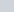. The drift velocity of electrons is of the order of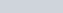. Drift velocity is proportional to electric current. If a material is resistive in nature then it is also proportional to the magnitude of an external electric field E, which is applied to the both end of the conductor.

It is proportional to the external electric field because due to the influence of this electric field all the free electrons tends to align into a particular direction and then, we get a net average velocity from the negative end to the positive end called drift velocity.

## DRIFT VELOCITY : DERIVATION

Let V is the potential difference of the power source, which applied across the ends of the conductor of the length, then the magnitude of the electric field inside the conductor is –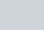The direction of the electric field is from positive to negative ends of the conductor. See figure below.

Since, we know that charge on each free electrons isand due to this all free electrons inside the conductor experience a electric force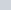.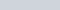Acceleration of each electrons is givens as-

(1)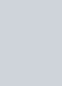At any instant of time, the velocity acquired by the electron having thermal velocity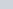is given by the first laws of motion is as follows –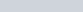Where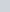is time elapsed. Similarly,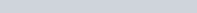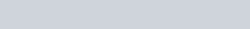Put the value of each thermal velocities in the formula of average velocity above-

(2)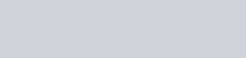We know that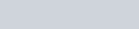and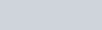is called relaxation time and it is denoted by. It’s value is of the order of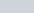seconds.Here, negative sign shows thatis opposite to the direction of the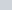.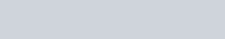## RELATION BETWEEN DRIFT VELOCITY AND ELECTRIC CURRENT

Higher the electric field, higher the drift velocity, higher the drift velocity higher the electric current. Electric current is proportional to the drift velocity. So we can relate the electric current to the drift velocity or vice versa.

Consider a conductor of lengthand cross sectional area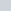. Therefore the volume of the conductor can be given as-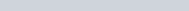If the conductor contains free electronper unit volume. Then the numbers of free electrons given as-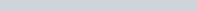Let e be the charge of an electron, then the total charge on all free electrons in the conductor is given by-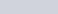Time taken by the free electrons to cover the length of the conductoris-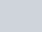Since, electric current is the rate of flow of charges, then-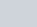Put the value of q and t in this equation,then we get-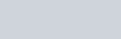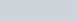This expression give the relationship between the drift velocity and electric current. If we put the value of drift velocity in this equation, then we get a expression of electric current in terms of relaxation time and electric field.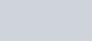## MOBILITY

Mobility is nothing but it is ability to move. Mobility tells us that how much free electrons are capable to move at per unit electric field.

It is defined as the magnitude of drift velocity per unit electric field.

It is expressed as –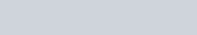Whereis the average relaxation time of the electrons while drifting towards the positive terminal /electrode and m is the mass of the free electrons/charged particles. It’s SI unit is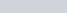or ms^{-1}N^{-1}C.

In a conductor, we know that electrons move towards the positive terminal or opposite to the direction of the electric field. When electrons move towards the positive terminal then the positive electron from the positive terminal move towards the negative terminal in order to neutralize the negative terminal.

But in any conductor, we know that there is only negatively charged free electrons. But from where these positive electrons came? In fact, there is not any physical existence of such positive electrons. It is just a hypothesis, which were given to support the conventional direction of electric current i.e. direction of motion of positive charge/positive electrons is the direction of electric current.

This positive electron is assumed as electron hole in the conductor. The number of these electron hole is same as the number of free electrons.

It is believed that when electron leaves its position then there exists a hole, which is positive in nature. In any conductor when electrons move towards the positive terminal then the electron hole move towards the negative terminal.

So here is two type of motion one motion is of electrons and second is the motion of positive electron/electron hole. Thus, the mobility of each particles is –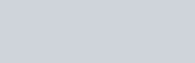Where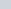andare the average relaxation time of electrons and holes respectively and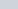and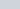refer to the mass of the electron and holes respectively. The magnitude of charge on both particles (electron and electron hole) is same i.e. electronic charge e. But holes have +e and electrons have -e charge.